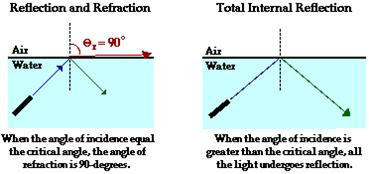## 2008-08-07

### Understanding Total Internal Reflection of Light

1. If the angle of incidence is allowed to exceed the critical angle, it is found that light rays are not refracted. This is because all of the light rays are reflected back.

2.This phenomenon is called total internal reflection.

3. Total Internal Reflection occurs when:
a. Light rays travel from a denser medium to a less dense medium.
b. The angle of incidence is greater than the critical angle.

Light ray which travels from a denser medium to a less dense medium will be refracted away from the normal.

Here are some Q and A session:Q: What happens when light passes from a transparent medium into air?

A: When light passes from a transparent medium into air, it bends away from the normal. It is refracted.Q: Why the angle of refraction becomes 90° and not more? What do we call the angle of incidence at this limit?

A: This is the limit the light ray can be refracted in air because the angle in air cannot be larger than 90°. The angle of incidence in the denser medium at this limit is called the critical angle, c.Q: What happens when the angle of incidence is more than the critical angle?

A: When the angle of incidence is greater than the critical angle, all the light undergoes reflection.

Later we will study the Relationship between Critical angle and Refractive Index